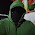# Kerala PSC - How to Calculate the Day of the week of any Given Date

How to Calculate the Day of the week of any given date in no time. It's a calendar calculation technique that I learned from a book. By Practicing it allows you to take a date, like 15 August 1947, and mentally calculate which day of the week it fell on.

Note : “Mod” means to divide the number and keep only the remainder. For Example if the Total of all codes is 58 mod 7, then divide 58/7 and keep only the reminder i.e. 2

The Year Code
To calculate the Year Code, use this formula:
YY is the last two digits of the year. For the year 1947, it’s 47.
First, divide YY by 4 and discard the remainder: 47 div 4 = 11.
Then add 11 back into the YY number, which is 47 in this case, resulting in 58.
The next step is: 58 mod 7. We’ve divided 58/7 and left with a remainder of 2. That is the Year Code for 1947.
Hold that in memory while you calculate the items below.

The Month Code
This is easy — just memorize the number 033614625035:
Memorize it as (033)(614)(525)(035)

The Century Code
Just remember the number 4206420:
(420)6(420)

Leap Year Code

Note : The other thing to take into account is whether you are dealing with a leap year. If the date is from January or February of a leap year, you have to subtract one from your total before the final step and for other months except Jan and Feb.

If you can divide a Gregorian year by 4, it’s a leap year, unless it’s divisible by 100. But it is a leap year if it’s divisible by 400.
1992 is a leap year because you can divide it by four.
1900 is not a leap year because you can divide it by 100.
2000 is a leap year because you can divide it by 400. Calculating the Day

Back to the original formula:
(Year Code + Month Code + Century Code + Date Number – Leap Year Code) mod 7
For 15 August 1947, here are the results:
Year Code: 2
Month Code: 2
Century Code: 0
Date Number: 15 (the 15th of the month)
Leap Year Code: 0
So:

(2 + 2 + 15) mod 7 = 19 mod 7 = 5

Match the resulting number in the list below, and you’ll have the day of the week:

0 = Sunday
1 = Monday
2 = Tuesday
3 = Wednesday
4 = Thursday
5 = Friday
6 = Saturday

15 August 1947 was on a Friday.

Leap Year Example :

Lets calculate the Day for 18 July 1996
Year Code : 1 (96/4=24+96=120, 120 Mod 7 = 1)
Month Code : 6
Century Code: 0
Date Number : 18
Leap Year Code : 0 (No minus because it doesn't fall in Jan and Feb)
So:
(1 + 6 + 18) mod 7 = 25 mod 7 = 4

18 July 1996 was on a Thursday

Another Leap Year Example :

1 January 2000: Start with ’00, leaving a Year Code of zero.
January has a Month Code of zero.
The Day Number is 1.
The Century Code for dates in the 2000s is 6.
2000 is a leap year, since it can be divided by 400, and the date is in a January or February, so subtract 1 from the total in the final step.
Answer: 0 + 0 + 1 + 6 – 1 = 6.

1 January 2000 was a Saturday

Today's
07 November 2017
Year Code : (17/4=leaving reminder we get 4) (4+17 Mod 7 = 0) because reminder is zero
Month Code : 3 (November)
Century Code : 6 (year falls in 2000 century)
Leap Year : 0 (No 2017 is not a leap year)
So.
(3 + 6 = 9 Mod 7 = 2)

Right Today is Tuesday

Hope you find this post useful,  If you have any questions, please leave a comment below.

1.2.1.